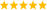LabVIEW

cancel
Showing results for
Did you mean:

Solve transcendental trig function for first solution?

I have searched the forums but have been unable to find a specific solution to this:

I need to solve for the first solution (first zero) to:

tan(c)=((a+b)*c)/((c^2)-ab); rearranged to:

c=atan(((a+b)*c)/((c^2)-ab)

I have tried the formula node, RIdder's and NR zero finder. Any help would be much appreciated.

Message 1 of 10
(1,043 Views)

Re: Solve transcendental trig function for first solution?

Both of the Zero Finder VIs throw errors: -23001 - Syntax error of parser.

1. These VIs do not have standard error out clusters. You must wire a numeric indicator to the error terminals to see the errors. I do not usually use automatic error handling, but I think it does not respond to this type or error indication.

2. It seems unlikely that c=atan(c) will be an acceptable formula with the same variable as both input and output.

3. Removing the "c=" part and just passing the right side expression eliminates the syntax error.

4. Similarly the formula node outputs are only values found on the left side of assignment statements. d = atan(c) with d as ipnut and c as output will not work.

5. Repeating the calculations in the for loops when none of the inputs changes between iterations does not make any sense.

6. Use wires rather than local variables.

Try transforming your equations so that the the output variable does not appear as an input. You may need to use a shift regsiter to assign the value of the output from iteration i as an input value for iteration i+1.

Lynn

Message 2 of 10
(1,025 Views)

Re: Solve transcendental trig function for first solution?

Thanks for the quick reply.

Per your suggestion, I have modified the formula by setting the original left side to "0", by moving "c" to the right, so all variables are on the right. I have also set the equation equal to "a", both formula nodes should give the same value for "c".

I'm unsure as to why both formulas output 0 as the value for "c". Shouldn't the value be similar to the Ridder or NR Zero?

Message 3 of 10
(1,017 Views)

Re: Solve transcendental trig function for first solution?

I have looked at things more carefully. The start - end range of 0 to 1 may be part of the problem. If you calculate the values of the formula over that range you find that the endpoints are very near zero. The curve starts at -0 and crosses zero near 0.5 and just before

1.

I changed the formula node so that the output variable to be d and the input variable to be c. I added a shift register for c. It starts at 0 and increments by 0.02 for 51 steps so that c covers 0 to 1 in increments of 0.02. The result looks like this:When I change start to 0.01 and end to 0.99 both zero finders return 0.50 as the zero location (although the f(zero) value is +/-pi/2).

Lynn

Message 4 of 10
(1,014 Views)

Re: Solve transcendental trig function for first solution?

Your formula nodes still have outputs as independent variables in the formulas. The upper formula node has no assignment statement. Thus, it has no output. The lower formula node assigns a value to a but not to c.

Both of the Zero finders return error -23021: Both function values have the same sign.  If you calculate the values of the formula at 0 and 1, both are negative. Plotting the formula over that input range shows a rising zero crossing near 0.16 and a falling zero crossing near 0.56. The zero finders appear to work well only when a single zero crossing occurs between the values of start and end.

Lynn

Message 5 of 10
(1,006 Views)

Re: Solve transcendental trig function for first solution?

Can you post your VI showing this? I'm missing something trying to recreate..

Message 6 of 10
(976 Views)

Re: Solve transcendental trig function for first solution?

Here is my revision to your VI.

1. I removed the local variables and connected the data with wires.

2. The formula node connected to "output variable" has no assignment statement.

3. The assignment statement in the lower formula node assigns a value to an input. The output variable does not have a value assigned.

4. I changed the start and end constants to controls and wired the values to both Zero Finder VIs.

5. I added the for loop with Eval Single-Variable Scalar.vi to calculate values for the formula. It is displayed on the XY Graph.

Note: being able to see the data on a graph can often suggest the nature of problems.

Lynn

Message 7 of 10
(949 Views)

Re: Solve transcendental trig function for first solution?

Hi Master.

Can you help me?

I need solver  transcendental equations in labview. Cotg (a) = a/ b

Thank you so much!

Message 8 of 10
(128 Views)

Re: Solve transcendental trig function for first solution?

(You probably should have started a new thread instead of adding to a 5 year old post of a different problem).

Well, did you study the above answers? How far did you get?

LabVIEW does not directly do any symbolic math, but as a start, type your function into wolfram alpha. Maybe it can give you some ideas.LabVIEW Champion. It all comes together inGCentralMessage 9 of 10
(107 Views)

Re: Solve transcendental trig function for first solution?

Thank altenbach So much!

I want use Partial Differential Equations VIs to define and solve a 1-dimensional heat equation in a rectangular domain.

At "Define boundary condition" , I don't know how to declare functions. Cotg (a) = a/ b

The program is reporting an error and does not produce results

Can you help me?

Thank you so much!

Message 10 of 10
(75 Views)